# Operational Amplifier

## Operational Amplifier

An operational amplifier (OP-Amp) is a multi-stage , direct coupled, high gain negative feedback amplifier that has one or more differential amplifiers and its concluded with a level translator and an output stage.A voltage-shunt feedback is provided in an op-amp to obtain a stabilized voltage gain.

Initially operational amplifier was used to perform  mathematical operations such as addition, subtraction, integration and differentiation. Nowadays , the application of op-amp’s varies from ac and dc signal amplification to use in active filters, oscillators, comparators, voltage regulators, instrumentation and control systems, pulse generators, square wave generators and many more electronic circuits.

### Block diagram of Operational Amplifier

Fig.1 shows the block diagram of an operational amplifier .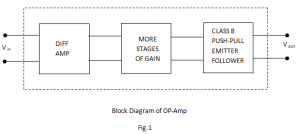An operational amplifier (OP-Amp) is a multistage amplifier and consists of three stages such as: differential amplifier (input stage) followed by a high gain CE amplifier stage and finally class B push-pull emitter follower as the output stage.

The key electronic circuit in an OP-AMP is the differential amplifier.

A differential amplifier (DA) can accept two input signals and amplifies the difference between these two input signals.

The following points may be noted about OP-Amps :

1. The input stage of an OP-Amp is a differential amplifier(DA) and the output stage is typically a class B push pull emitter follower.
2. The internal stages of an OP-Amp are direct coupled i.e. no coupling capacitors are used. The direct coupling allows the OP- Amp to amplify d.c as well as a.c. signals.
3. An OP-Amp has very high input impedance (ideally infinite) and very low output impedance (ideally zero). The effect of high output impedance is that the amplifier will draw a very small current (ideally zero) from the signal source. The effect of very low output impedance is that the amplifier will provide a  constant output voltage independent of current drawn from the source.
4. An OP-Amp has very high open loop voltage gain (ideally infinite); typically more than 200000.
5. The OP-Amps are almost always operated with negative feedback. It is because the open loop voltage gain of these amplifiers is very high and we can sacrifice the gain to achieve the advantages of negative feedback including large bandwidth (BW) and gain stability .

### Schematic symbol of Operational Amplifier

Fig.2 shows the schematic symbol of an operational amplifier .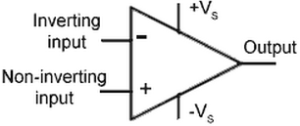Fig.2

The basic operational amplifier has five terminals: two high impedance inputs, one called the inverting input, marked with a negative sign (-) and the other one called non-inverting input, marked with a positive sign (+), two terminals for supply voltage +Vand -V and one output terminal.

A signal applied to + terminal will appear in the same phase at the output as at the input.

A signal applied to – terminal will be shifted in phase 180at the output.

### A.C. Analysis of  Operational Amplifier

Practical OP-Amp:

Fig.3 shows the equivalent circuit of a practical  OP-Amp.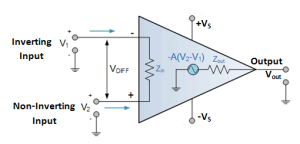Fig.3

The input voltage difference V2 -Vappears between the two input terminals and the output voltage  is -A(V2 -V1) taken through the output impedance Zout.

Since the voltage gain A of a practical OP-Amp is very high, an extremely small input voltage (V2 -V1) will produce a large output(Vout).

Since the input impedance, Zin  is very high, a practical OP-Amp has very small input current.

Since the output impedance, Zout is very low, the output voltage is practically independent of the value of load connected to OP-Amp.

### Ideal OP-Amp

Fig.4 shows the a.c. equivalent circuit of an ideal OP-Amp.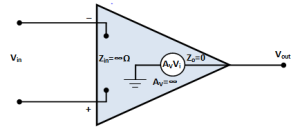Fig.4

The characteristics of an ideal OP-Amp are:

1. It has infinite voltage gain
2.  Infinite input impedance
3. Zero output impedance

The consequence of these properties of an ideal OP-Amp are:

1.  Since the voltage gain Aof  an ideal OP-Amp is infinite, it means that we can set Vin=0V.
2. Since the input impedance Zin is infinite, an ideal OP-Amp has zero input current.
3. Since the output impedance Zout is zero, the output voltage does not depends on the value of load connected to OP-Amp.

### Bandwidth of an Operational Amplifier

All electronic devices work only over a limited range of frequencies. This range of frequencies is called bandwidth.

Every OP-Amp has a bandwidth i.e. the range of frequencies over which it will work properly.

The bandwidth of an OP-Amp depends upon he closed-loop gain of the OP-Amp circuit.

One important parameter is gain-bandwidth product (GBW). It is defined as: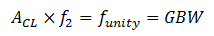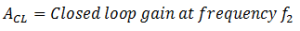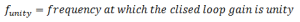It can be proved that the gain-bandwidth product of an OP-Amp is constant. Since an OP-Amp is capable of operating as d.c. amplifier, its bandwidth is (f2 – 0). The gain-bandwidth product of an OP-Amp is an important parameter because it can be used to find:

• The maximum value of ACL at a given value of f2.
• The value of f2 for a given value of ACL.

Slew Rate

The slew rate of an OP-Amp is a measure of how fast the output voltage can change and its measured in volts per microsecond (V/µs).

Since frequency is a function of time, the slew rate can be used to determine the maximum operating frequency of the OP-Amp as follows:

Maximum operating frequency,

Here, Vpk is the peak output voltage.

### OP-Amp with Negative Feedback

An OP-Amp is almost always operated with negative feedback i.e. part of the output is fed back in phase opposition to the input.Fig.5

The reason is as follows:

The open-loop voltage gain of an OP-Amp is very high (usually greater than 100,000). Therefore, an extremely small input voltage drives the OP-Amp into its saturated output stage.

For example, assume Vin = 1m V and AOL = 100,000

Then, Vout = AOL vin= (100,000)× (1m V) = 100 V

Since the output level of an OP-Amp can never reach 100 V, it is driven deep into saturation and the device becomes non-linear.

With negative feedback, the voltage gain (ACL) can be reduced and controlled so that OP-Amp can function as a linear amplifier.

In addition to providing a controlled and stable gain, negative feedback also controls the input and output impedance and amplifier bandwidth.